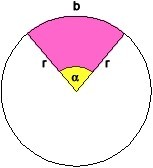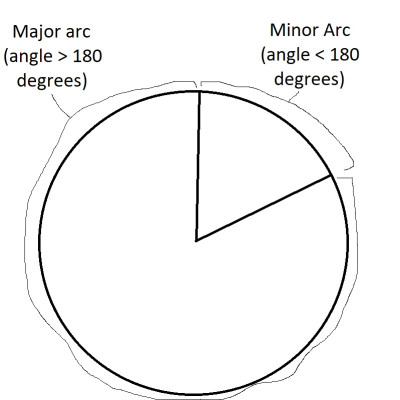# Arc Length Of A Circle (3 Key Concepts To Grasp)

You might be familiar with finding the circumference (perimeter) of a circle.  However, finding the arc length takes an extra step, since we are finding just a part of the circumference.

So, what do you need to know about the arc length of a circle?  The arc length S of a circle is S = Rθ, where R is the radius and θ is the angle measure of the arc (in radians). The equivalent formula is S = πRθ/180 if θ is in degrees. Arc length is a percentage of the entire circumference, based on the ratio of the angle θ over 2π radians (360 degrees).

Of course, there are various situations when we might need to calculate arc length, including minor, semicircular, and major arcs.

In this article, we’ll take a closer look at arc length of a circle and what it means.  We’ll also look at some examples of how to calculate the arc length of a circle in various cases.

Let’s get started.

## Arc Length Of A Circle

The arc length of a circle is a part of the circumference.  It corresponds to the angle measure of the circular sector.The arc length of a circle is a part of the circumference. It depends on the radius of the circle and the angle measure of the sector.

One way to think of arc length is how far you would have to walk to go around a part of the circle.  The arc length of an entire circle would be the whole circumference, while the arc length for a semicircle would be half of the circumference.

### Is Arc Length The Same As Circumference?

Arc length is not the same as circumference.  However, the concepts are related.

The circumference of a circle is the arc length of the entire circle (that is, a sector with an angle measure of 2π radians or 360 degrees).

Any other arc length is a fractional part of the arc length.  The fraction is based on the angle measure of the specific arc, divided by 2π radians (or 360 degrees).The arc length of a circle is a part of the circumference. The part is a fraction that depends on the angle measure.

### Arc Length Formula For A Circle

The arc length formula for a circle depends on whether the angle measure of the arc is given in radians or degrees.

• S = Rθ, where S is arc length, R is radius of the circle, and θ is the angle measure of the arc in radians.

Arc Length Formula Degrees:

• S = πRθ/180, where S is arc length, R is radius of the circle, and θ is the angle measure of the arc in degrees.

We can derive each of these formulas if we remember the key fact about arc length of a circle:

The arc length of a circle is a fraction of the total circumference.  This fraction is the angle measure of the arc divided by the entire circle’s measure (either 2π or 360 degrees).

First, let’s derive the formula for arc length of a circle in radians.  Our formula is:

• (Arc Length) = [(Arc Angle Measure) / 2π]*(Circumference of Circle)
• (Arc Length) = [θ / 2π]*(Circumference of Circle)
• (Arc Length) = [θ / 2π]*(2πR)
• (Arc Length) = [2πRθ / 2π]
• (Arc Length) = [Rθ / 1]
• S = Rθ

Now, let’s derive the formula for arc length of a circle in degrees.  Once again, our formula is:

• (Arc Length) = [(Arc Angle Measure) / 2π]*(Circumference of Circle)
• (Arc Length) = [θ / 360]*(Circumference of Circle)
• (Arc Length) = [θ / 360]*(2πR)
• (Arc Length) = [2πRθ / 360]
• (Arc Length) = [πRθ / 180]
• S = πRθ / 180

### What Are The Types Of Arcs?

There are three main types of arcs that we will come across when measuring arc length:

• Minor Arc – this is an arc with an angle measure less than 180 degrees (π radians), but greater than or equal to 0 degrees (0 radians).
• Semicircular Arc – this is an arc with an angle measure equal to 180 degrees (2π radians).
• Major Arc – this is an arc with an angle measure greater than 180 degrees (π radians), but less than or equal to 360 degrees (2π radians).

The minor arc and major arc of a circle add up to 360 degrees; that is:

• (Angle Measure of Minor Arc) + (Angle Measure of Major Arc) = 360The minor and major arcs of a circle add up to 360 degrees. The minor arc is less than 180 degrees, and the major arc is greater than 180 degrees.

Their total arc length is equal to the entire circumference of the circle.

Also, two semicircular arcs in a circle add up to 360 degrees.

Likewise, their total arc length is equal to the entire circumference of the circle.

### How Do You Find Arc Length?

To find arc length, there are three basic steps:

• Step 1: Find the circumference C of the circle with the formula C = 2πR.
• Step 2: Find the fraction θ/2π (radians) or θ/360 (degrees).
• Step 3: Find the product of steps 1 and 2.

Alternatively, we can use the shortcut formulas given above.  Just make sure to use the right formula, depending on whether the angle measure is given in radians or degrees!

#### Example 1: Finding A Minor Arc Length (Radians)

Let’s say that you have a circle with a radius of 9 feet (so R = 9).

You want to find the arc length of the minor arc with angle measure π/3 (so θ = π/3).

We will use the formula for arc length of a circle given an angle in radians:

• S = Rθ

We already know that R = 9 feet and θ = π/3, so we get:

• S = 9(π/3)
• S = 3π

The arc length is 3π feet, or approximately 9.42 feet.

Note that this arc length is 1/6 of the circumference, since C = 2πR = 2π(9) = 18π.

#### Example 2: Finding A Minor Arc Length (Degrees)

Let’s say that you have a circle with a radius of 10 feet (so R = 10).

You want to find the arc length of the minor arc with angle measure 45 degrees (so θ = 45).

We will use the formula for arc length of a circle given an angle in degrees:

• S = πRθ / 180

We already know that R = 10 feet and θ = 45, so we get:

• S = πRθ / 180
• S = π(10)(45) / 180
• S = 450π / 180
• S = 5π / 2
• S = 2.5π

The arc length is 2.5π feet, or approximately 7.85 feet.

Note that this arc length is 1/8 of the circumference, since C = 2πR = 2π(10) = 20π.

#### Example 3: Finding A Semicircular Arc Length

Let’s say that you have a circle with a radius of 4 feet (so R = 4).

You want to find the arc length of a semicircular arc.  So the angle measure is π radians or 180 degrees.

We will use the formula for arc length of a circle given an angle in radians:

• S = Rθ

We already know that R = 4 feet and θ = π, so we get:

• S = 4(π)
• S = 4π

The arc length is 4π feet, or approximately 12.57 feet.

Note that this arc length is 1/2 of the circumference, since C = 2πR = 2π(4) = 8π.

(We could also use the arc length formula for degrees with θ = 180 to get the same result).

#### Example 4: Finding A Major Arc Length (Radians)

Let’s say that you have a circle with a radius of 12 feet (so R = 12).

You want to find the arc length of the minor arc with angle measure 5π/4 (so θ = 5π/4).

We will use the formula for arc length of a circle given an angle in radians:

• S = Rθ

We already know that R = 12 feet and θ = 5π/4, so we get:

• S = 12(5π/4)
• S = 60/4
• S = 15π

The arc length is 15π feet, or approximately 47.12 feet.

Note that this arc length is 5/8 of the circumference, since C = 2πR = 2π(12) = 24π.

#### Example 5: Finding A Major Arc Length (Degrees)

Let’s say that you have a circle with a radius of 15 feet (so R = 15).

You want to find the arc length of the minor arc with angle measure 210 (so θ = 210).

We will use the formula for arc length of a circle given an angle in degrees:

• S = πRθ/180

We already know that R = 15 feet and θ = 6π/5, so we get:

• S = π(15)(210)/180
• S = 17.5π

The arc length is 17.5π feet, or approximately 54.98 feet.

Note that this arc length is 7/12 of the circumference, since C = 2πR = 2π(15) = 30π.

## Conclusion

Now you know what the arc length of a circular sector is and how to find it.  You also know about minor, semicircular, and major arcs, along with the differences between them.

You can learn about the area of a circular sector here.

You can learn about uses of circles here.

You can learn about the history of pi and how it is connected to circles here.